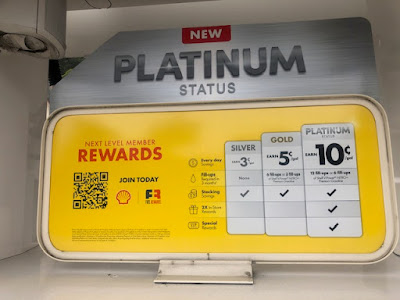## March 02, 2023

### Statistical Significance of A/B Tests

There is a ton of misinformation out there about A/B testing. Those lacking rigorous statistical training tell you that you need "x" responses for a valid A/B test.

That's not how this stuff works.

More than thirty years ago (Lands' End), we developed an equation to estimate the variance associated with our A/B mailing tests. It turned out that the variance of our estimates was non-constant. In other words, the variance might be "x" when the dollar-per-book was \$3.00 ... it might be "1.5x" at a dollar per book of \$5.00.

We developed an equation ... as long as dollars-per-book was >= \$2.00 variance could be estimated as -188 + 192*x, where "x" was the dollars-per-book in a test group (aside ... there are going to be statistical experts who balk at creating this equation ... one that accounts for non-constant variance ... and will say that everything that follows is garbage ... just want you to know that view is out there, I need to be forthright here).

Let's pretend that our control dollar-per-book was \$3.00, and we expected the test dollar-per-book to be \$3.25. Let's pretend that we wanted 10,000 customers in the test group and 10,000 customers in the control group. Would our results be statistically significant?

The t-test equation looked like this:

• Test Group Dollar-Per-Book = \$3.25.
• Control Group Dollar-Per-Book = \$2.75.
• Test Group Sample Size = 10,000.
• Control Group Sample Size = 10,000.
• Variance of Test Group = -188 + 192*3.25 = 436.
• Variance of Control Group = -188 + 192*3.00 = 388.
• T-Score = (3.25 - 3.00) / SQRT(436/10000 + 388/10000) = (0.25) / (0.29) = 0.86.

The T-Score is nowhere close to 2.00 ... so the results are not statistically significant.

Now, does that mean that the results aren't meaningful? Maybe. What would happen if we had 100,000 customers in each of the test/control group?

• T-Score = (3.25 - 3.00) / SQRT(436/100000 + 388/100000) = (0.25) / (0.09) = 2.78.

Now the results are statistically significant.

What was the difference?

Well, the original sample size was too small.

We used the equation above to determine the appropriate sample size for all tests based on the amount of variance associated with our expectations for test group performance and holdout group performance.

When I worked at Nordstrom, we used a comparable equation - one specific to Nordstrom. We learned that we needed 100,000 or 200,000 customers to measure what we wanted to measure ... not 10,000 or 20,000.

Comparable issues impact website conversion. You don't want to measure conversions ... you want to measure sales per visitor. Your test group might spend more/less than the control group once the decision to purchase is made ... so you have to measure sales per visitor.